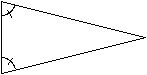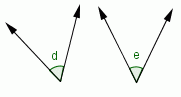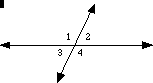Email us to get an instant 20% discount on highly effective K-12 Math & English kwizNET Programs!

#### Online Quiz (WorksheetABCD)

Questions Per Quiz = 2 4 6 8 10

### MEAP Preparation - Grade 7 Mathematics4.5 Adjacent, Congruent, Linear Pair, and Vertically Opposite Angles

 Adjacent Angles: Two angles in a planes are called adjacent angles, if they have a common vertex, a common side, and their interiors do not have a common point. �1 and �2 below are adjacent angles.Congruent Angles: Angles having the same measure are called congruent angles. The two marked angles in the following isosceles triangle are congruent angles and �d and �e are congruent.Linear Pair of Angles: A pair of adjacent angles formed by intersecting lines is called a Linear Pair of Angles. In the following picture, �1 & �2, �2 & �4, �3 & �4, and �3 & �4 are linear pairs.Vertically Opposite Angles: When two lines intersect, then the angles that are opposite one another at the intersection are called Vertically Opposite Angles. The measures of the vertically opposite angles are equal. In the above picture, �1 & �4, and �2 & �3 are vertically opposite. Directions: Answer the following questions. Also draw all the above kinds of angles, measure them using protractor and label them.
 Q 1: Any two angles having a common vertex and a common arm are adjacent.FalseTrue Q 2: Any two right angles are supplementary.TrueFalse Q 3: Any two adjacent angles have a common vertex.FalseTrue Q 4: If two lines are perpendicular to each other, then all the pairs of vertically opposite angles formed are supplementary.TrueFalse Q 5: Any two supplementary angles are coplanar.FalseTrue Q 6: If two angles are congruent, they are supplementary.FalseTrue Q 7: If two lines are perpendicular to each other then all the pairs of adjacent angles formed are supplementary.TrueFalse Q 8: Any two complementary angles are adjacent.TrueFalse Question 9: This question is available to subscribers only! Question 10: This question is available to subscribers only!